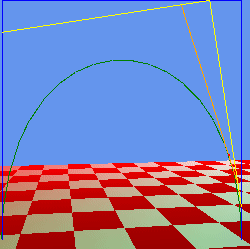# Curved, bezier lines in Monogame 3.8?

Hi

Anyone knows how to Draw - curved lines in Monogame?
In Extended there is the DrawLine and via Primitives2D there is the DrawArc, but no curved lines is possible?

And a bonus-question: How to fill up a polygon?
If I use the DrawPolygon there is no functionality for fill, only thickness of the polygone-line

Thanks!

Interesting, DrawLine is not implemented…

EDIT

Oh, interesting, I looked at the code and it looks like a custom implementation!

if its just for simple testing you can just use spritebatch to draw lines using regular old draw.

``````public static void DrawBasicLine(Vector2 s, Vector2 e, int thickness, Color linecolor)
{
spriteBatch.Draw(dot, new Rectangle((int)s.X, (int)s.Y, thickness, (int)Vector2.Distance(e, s)), new Rectangle(0, 0, 1, 1), linecolor, (float)Atan2Xna(e.X - s.X, e.Y - s.Y), Vector2.Zero, SpriteEffects.None, 0);
}
public static float Atan2Xna(float difx, float dify)
{
if (SpriteBatchAtan2)
return (float)System.Math.Atan2(difx, dify) * -1f;
else
return (float)System.Math.Atan2(difx, dify);
}
``````

A curve is just a series of lines if you need to draw a curve faster dynamically.
You’ll probably need to do so on a shader or something that’s a pretty complicated topic.
Statically you can use the line strategy and buffer the lines into a vertice buffer.

Varying the time below will give you points on the curve to use to form line segments.
You could put this onto a shader rendering a quad around the drawing area.

``````public Vector3 CalculateBspline(Vector4 a0, Vector4 a1, Vector4 a2, Vector4 a3, float time)
{
var a4 = (a1 - a0) * time + a0;
var a5 = (a2 - a1) * time + a1;
var a6 = (a3 - a2) * time + a2;
var a7 = (a5 - a4) * time + a4;
var a8 = (a6 - a5) * time + a5;
var a9 = (a8 - a7) * time + a7;
solved = a0;
solved = a1;
solved = a2;
solved = a3;
solved = a4;
solved = a5;
solved = a6;
solved = a7;
solved = a8;
solved = a9;
}
``````

This is a incomplete sort of test project i started not sure were i was going with it really but game2 i believe will draw a simple subdivision bezier b-spline.3 Likes

if you can use external libraries, you could check this too:

2 Likes

Also look for this https://github.com/DoogeJ/MonoGame.Primitives2D

It expands spritebatch to draw 2d primitives and you can easily expand it to do other 2d shapes

1 Like